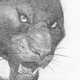# Could a tabletop experiment detect gravitational waves and determine the quantum nature of gravity?

Perhaps the most surprising prediction of general relativity is that of gravitational waves. Ripples in space and time that spread through the universe at the speed of light. Gravitational waves are so faint that for decades their detection was thought impossible. Even today, it takes an array of laser interferometers several kilometers long to see their effect. But what if we could detect them with a table-top experiment in a university lab?

In a recent paper published in the New Journal of Physics, a team of physicists proposes just such a device. Rather than using beams of light, they suggest using the quantum superposition of a single electron.

Their design uses a tiny diamond where one of the carbon atoms is replaced with a nitrogen atom. This would create a gap within the diamond where you can place an extra electron. Since electrons have a rotation-like property known as spin, this electron has two possible orientations or states. By shining a beam of laser light on the diamond, you could put the electron in a superposition of both states.

Superposition is one of the weird aspects of quantum theory, where an object can be in an indefinite combination of two outcomes. It is as if a flipped coin was neither heads nor tails, but a fuzzy state of either. The coin would be in a superposition of both possibilities. For the electron, it would be in a superposition of its two possible spin states.

Even when the electron is in this fuzzy state, it can still interact with other things. So the team proposes placing the diamond in a magnetic field, which would cause the superposition to skew. The two possible spin states would shift, with one moving toward the north magnetic pole, and one moving toward the south magnetic pole. As a result, the superposition splits into two regions. If a gravitational wave were to pass by, each part of the superposition would be affected differently. By measuring this difference, you could detect the passing of a gravitational wave.

To go back to our coin analogy, It would be like taking the fuzzy heads-or-tails state and separating the potential heads from the potential tails, then recombining them and measuring the outcome of the coin toss. If nothing interacts with the coin, your results would be heads half the time and tails the other half. But if a gravitational wave interacted with them, then your outcome wouldn’t be 50/50. It might be slightly more likely tails than heads.

This experiment would be challenging to perform. You would need to shield it from any outside electrical interference, and you would also need to do it in free fall. So it would either have to be performed in orbit, or while being dropped down a long shaft. It could be decades before such an experiment could be done. But the team has shown that this kind of experiment could work in principle. It would be sensitive enough to measure gravitational waves. It could also study an aspect of gravity LIGO never will.

Although general relativity is a robust scientific theory, describing everything from planetary orbits to black holes, it breaks down at microscopic scales. Quantum theory, which correctly describes atoms and molecules’ behavior, directly contradicts the tenets of general relativity. We think there is a more profound theory that combines these two theories. We call this the theory of quantum gravity. We don’t know how quantum gravity works, but that could change with this new experiment. As the team points out, it would be not only capable of detecting gravitational waves but also detecting other effects of general relativity on atomic scales. It would be one of our first experimental looks at quantum gravity.

Reference: Marshman, Ryan James, et al. “Mesoscopic Interference for Metric and Curvature (MIMAC) & Gravitational Wave Detection.” New Journal of Physics (2020).

## One Reply to “Could a tabletop experiment detect gravitational waves and determine the quantum nature of gravity?”

1.Torbjörn Larsson says:

“Quantum theory, which correctly describes atoms and molecules’ behavior, directly contradicts the tenets of general relativity.”

Other than the sense that a classical theory which, say, hasn’t superposition of single particles do, can you give an example? I don’t think there is one, or relativity wouldn’t play nice with quantum mechanics in quantum field theory (and they wouldn’t both break down at the same energy density of Planck energy).